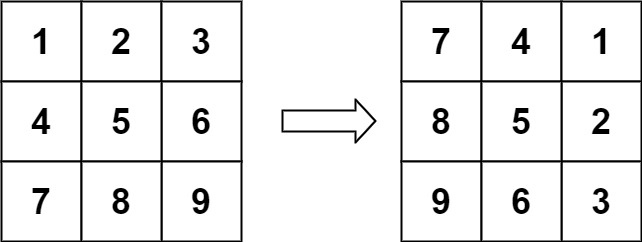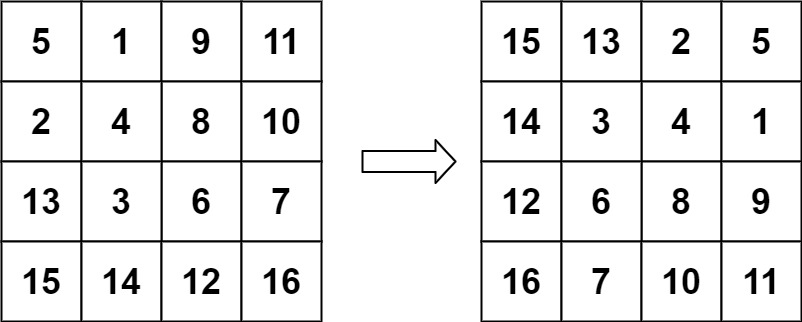0048. 旋转图像

# 0048. 旋转图像#

• 标签：数组
• 难度：中等

## 题目大意 #

• 不能使用额外的数组空间。
• $n == matrix.length == matrix[i].length$。
• $1 \le n \le 20$。
• $-1000 \le matrix[i][j] \le 1000$。

• 示例 1：1 2  输入：matrix = [[1,2,3],[4,5,6],[7,8,9]] 输出：[[7,4,1],[8,5,2],[9,6,3]] 
• 示例 2：1 2  输入：matrix = [[5,1,9,11],[2,4,8,10],[13,3,6,7],[15,14,12,16]] 输出：[[15,13,2,5],[14,3,4,1],[12,6,8,9],[16,7,10,11]] 

## 解题思路 #

### 思路 1：原地旋转 #

matrixnew[j][n - i - 1] 的点经过旋转移动到了 matrix[n − i − 1][n − j − 1] 的位置。

matrix[n − i − 1][n − j − 1] 位置上的点经过旋转移动到了 matrix[n − j − 1][i] 的位置。

matrix[n− j − 1][i] 位置上的点经过旋转移动到了最初的 matrix[i][j] 的位置。

### 思路 1：代码 #

 1 2 3 4 5 6 7  class Solution: def rotate(self, matrix: List[List[int]]) -> None: n = len(matrix) for i in range(n // 2): for j in range((n + 1) // 2): matrix[i][j], matrix[n - j - 1][i], matrix[n - i - 1][n - j - 1], matrix[j][n - i - 1] = matrix[n - j - 1][i], matrix[n - i - 1][n - j - 1], matrix[j][n - i - 1], matrix[i][j] 

### 思路 1：复杂度分析 #

• 时间复杂度：$O(n^2)$。
• 空间复杂度：$O(1)$。

### 思路 2：代码 #

  1 2 3 4 5 6 7 8 9 10  def rotate(self, matrix: List[List[int]]) -> None: n = len(matrix) for i in range(n // 2): for j in range(n): matrix[i][j], matrix[n - i - 1][j] = matrix[n - i - 1][j], matrix[i][j] for i in range(n): for j in range(i): matrix[i][j], matrix[j][i] = matrix[j][i], matrix[i][j] 

### 思路 2：复杂度分析 #

• 时间复杂度：$O(n^2)$。
• 空间复杂度：$O(1)$。